# Bayes' theorem facts for kids

Kids Encyclopedia Facts

In probability theory and applications, Bayes' theorem shows the relation between a conditional probability and its reverse form. For example, the probability of a hypothesis given some observed pieces of evidence, and the probability of that evidence given the hypothesis. This theorem is named after Thomas Bayes (how to say: /ˈbeɪz/ or "bays") and is often called Bayes' law or Bayes' rule.

## Formula

The equation used is: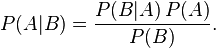$P(A|B) = \frac{P(B | A)\, P(A)}{P(B)}.$

Where:

• P(A) is the prior probability or marginal probability of A. It is "prior" in the sense that it does not take into account any information about B.
• P(A|B) is the conditional probability of A, given B. It is also called the posterior probability because it is derived from (or depends upon) the specified value of B.
• P(B|A) is the conditional probability of B given A. It is also called the likelihood.
• P(B) is the prior or marginal probability of B, and acts as a normalizing constant.

In many scenarios, P(B) is calculated indirectly using the formula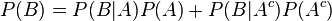$P(B) = P(B|A)P(A) + P(B|A^c)P(A^c)$, which simply states that the probability of B is the sum of the conditional probabilities based on whether A has occurred or not.

## Example

A simple example is as follows: There is a 40% chance of rain on Sunday. If it rains on Sunday, there is a 10% chance of rain on Monday. If it did not rain on Sunday, then there is an 80% chance that it will rain on Monday.

"Raining on Sunday" is event A, and "Raining on Monday" is event B.

• P( A ) = 0.40 = Probability of rain on Sunday.
• P( A ) = 0.60 = Probability of no rain on Sunday.
• P( B | A ) = 0.10 = Probability of rain on Monday, if it rained on Sunday.
• P( B | A ) = 0.90 = Probability of no rain on Monday, if it rained on Sunday.
• P( B | A ) = 0.80 = Probability of rain on Monday, if it did not rain on Sunday.
• P( B |A` ) = 0.20 = Probability of no rain on Monday, if it did not rain on Sunday.

The first thing we would normally calculate is the probability of it raining on Monday: This would be the sum of the probability of "Rain on Sunday and rain on Monday" and "No rain on Sunday and rain on Monday":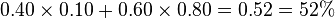$0.40\times0.10 + 0.60\times0.80 = 0.52 = 52%$ chance

However, we were asked to calculate the probability that it rained on Sunday, given that it rained on Monday, then this is where Bayes' theorem comes in. It allows us to calculate the probability of an earlier event, given the result of a later event.

The equation used is:$P(A|B) = \frac{P(B | A)\, P(A)}{P(B)}.$

In our case, "Rain on Sunday" is event A, and "Rain on Monday" is event B.

• P(B|A) = 0.10 = Probability of rain on Monday, if it rained on Sunday.
• P(A) = 0.40 = Probability of rain on Sunday.
• P(B) = 0.52 = Probability of rain on Monday.

So, to calculate the probability it rained on Sunday, given that it rained on Monday, we use the formula:$P(A|B) = \frac{P(B | A)\, P(A)}{P(B)}.$

or: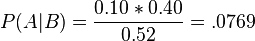$P(A|B) = \frac{0.10*0.40}{0.52} = .0769$

In other words, if it rained on Monday, then there is a 7.69% chance it rained on Sunday.

## Intuitive explanation

To calculate the probability of it having rained on Sunday, given that it rained on Monday, we can take the following steps:

• We know that it rained on Monday. Therefore, the total probability is P(B).
• The probability it rained on Sunday is P(A).
• The probability it rained on Monday, given that it rained on Sunday is P(B|A).
• The probability of raining on Sunday and raining Monday is P(A)*P(B|A).
• Therefore, the total probability of it having rained on Sunday, given that it rained on Monday, is the chance of it raining on Sunday and Monday divided by the total chance of it having rained on Monday.

Therefore,$P(A|B) = \frac{P(B | A)\, P(A)}{P(B)}.$

Another way to see this, which shows where Bayes' theorem comes from, is to consider the probability P(AB) that it rains on both Sunday and Monday. This can be calculated in two different ways, which give the same answer for P(AB):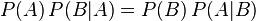$P(A)\, P(B|A) = P(B)\, P(A|B)$

In this regard, Bayes' theorem is just another way to write that equation.

## Images for kidsBayes' theorem Facts for Kids. Kiddle Encyclopedia.